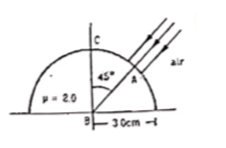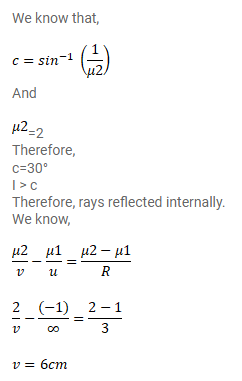# Figure shows a transparent hemisphere ofQuestion:

Figure shows a transparent hemisphere of radius $3.0 \mathrm{~cm}$ made of a material of refractive index $2.0$

(a) A narrow beam of parallel rays is incident on the hemisphere as shown in the figure. Are the rays totally reflected at the plane surface?

(b) Find the image formed by the refractive index at the first surface.

(c) Find the image formed by the reflection or by the refraction at the plane surface.

(d) Trace qualitatively the final rays as they come out the hemisphere.Solution: